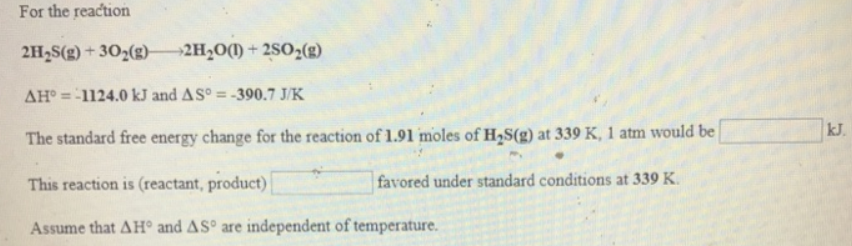# Problem: For the reaction 2H2S (g) + 3O2 (g) → 2H20 (l) + 2SO2 (g)  ΔH° = -1124.0 kJ and ΔS° = -390.7 J/K The standard free energy change for the reaction of 1.91 moles of H2S(g) at 339 K, 1 atm would be ________ kJ.This reaction is (reactant, product) _________ favored under standard conditions at 339 K.Assume that ΔH° and ΔS° are independent of temperature.

###### FREE Expert Solution
80% (449 ratings)###### Problem Details

For the reaction

2H2S (g) + 3O2 (g) → 2H20 (l) + 2SO2 (g)

ΔH° = -1124.0 kJ and ΔS° = -390.7 J/K

The standard free energy change for the reaction of 1.91 moles of H2S(g) at 339 K, 1 atm would be ________ kJ.

This reaction is (reactant, product) _________ favored under standard conditions at 339 K.

Assume that ΔH° and ΔS° are independent of temperature.